Next: BSpline interpolation Up: Interpolation Previous: Interpolation

### Quaternion interpolation

For various reasons, interpolation rotation with Euler angles is less than ideal. A naive approach to rotational interpolation could be to simply interpoloate between each Euler angle to produce inbetween frames. But the motion specified by such a rotation is oftentimes jerky and ill specified. This is illustrated by there not being a unique path between every two orientations across different coordinate systems. For these reasons quaternion interpolation of the rotational parameters is performed. Interpolating in quaternion space ensures a unique path under all circumstances.

Since Euler angles present a more agreeable user interface, the process of quaternion interpolation starts by generating a rotation matrix R from a set of Euler angles. This matrix is then lifted into quaternion space where the rotation is specified by a quaternion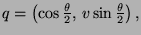where v specifies an axis of rotation and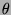the angle by which to rotate around the axis. Note that q is a unit quaternion, so the set of rotation matrices corresponds to the set of quaternions on the unit hypersphere.

Rotation can then be carried out directly in quaternion space by encoding a point to be rotated as a pure quaternion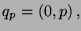and producing a rotated pure quaternion qr by qr=qqpq-1. It is oftentimes beneficial to concatenate other transformations along with rotations however, so after interpolation q is transformed back into a rotation matrix.

Since the set of quaternions that specify rotations live on the unit hypersphere, a direct interpolation between quaternions does not produce the desired result, as interpolants would stray from the hypersphere. Instead a spherical interpolation is performed. This procedure is outlined in [watt92].

spherical interpolation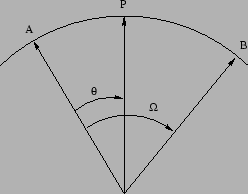The function slerp does a spherical linear interpolation between two quaternions A and B by an amount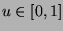: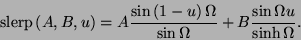To achieve C2 continuity between curve segments, a cubic interpolation must be done. In quaternion space this is somewhat complicated. The method used is to compose a cubic interpolation as a set of three linear interpolations. First between the data points and two other (carefully chosen) points, and then between the remaining points by an amount specified by the logistic equation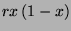with r=2. If the auxiliary points are chosen properly, then C2 continuity can be ensured. The function squad does a cubic interpolation between data points b0 and b3 by an amount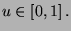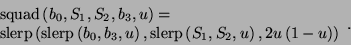The points S1 and S2 are called inner quadrangle points, and have to be chosen carefully so that continuity is guaranteed across segments. Given a quadrangle of quaternions,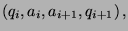where qi and qi+1 are our data points, we can guarantee C2 continuity by choosing ai and ai+1 as follows: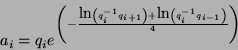We can summarize the process of interpolating between two rotation matrices as follows

interpolate_rotation(mat[n],t)

t_int = int(t);

t_fract = t - t_int;

q_0 = mat2quat(mat[t_int-1]);

q_1 = mat2quat(mat[t_int]);

q_2 = mat2quat(mat[t_int+1]);

q_3 = mat2quat(mat[t_int+2]);

q_i = slerp(slerp(q_1, q_2, t_fract),

slerp(i_1, i_2, t_fract),

2*t_fract*(1-t_fract);

return mat2quat(q_i);Next: BSpline interpolation Up: Interpolation Previous: Interpolation
brian martin
1999-06-23